# Game Development – A* on Triangulated Spaces: Part 2

| 5 min. (931 words)

In this post I’m going to cover how to implement A* on a triangulated graph with accurate best paths.

In the previous post I used a native path length estimation using the centre of each triangle to calculate distances between triangles and heuristics. This doesn’t work out for a couple reasons.

Primarily it overestimates the distance between two triangles, this compounds – meaning paths with less triangles are generally preferred over paths with many triangles. This is especially harmful when the optimal path should consist of many small triangles instead of a few large triangles. The simplification also breaks one of the primary assumptions of A* – that the path length function is accurate.

Secondly, it means the calculated heuristic between any node and the goal node is no longer admissible. One would assume that the distance between two triangles should be calculated by the closest point between each instead of their centre points. This leads to large triangles being judged as having higher heuristic values than smaller ones, because of the greater discrepancy between their centre and edge points.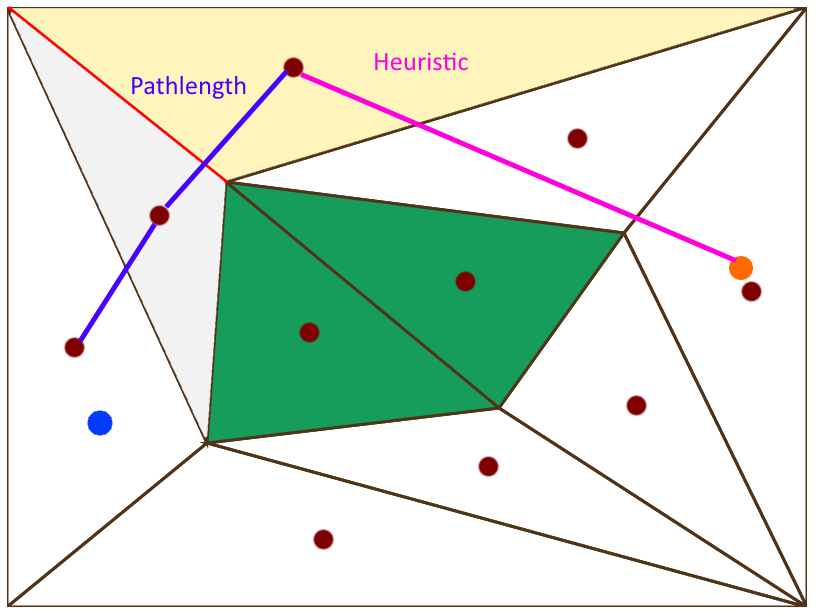Pathlength and heuristic of the yellow triangle using naive estimations.

To resolve these issues, a better system of heuristics and path length calculations is required. Luckily, many alternatives have been forwarded. A version of A* named Triangular A* (TA) is defined in a paper by Douglas Jon Demyen with several modifications to path length and heuristic calculation which results in an implementation of A on a triangulated space, which produces optimal paths in many cases.

Improving the heuristic calculation of the distance to the goal is simple. The shortest distance between a triangle’s edges and the goal point produces an admissible heuristic (no potential real path can be shorter than this heuristic).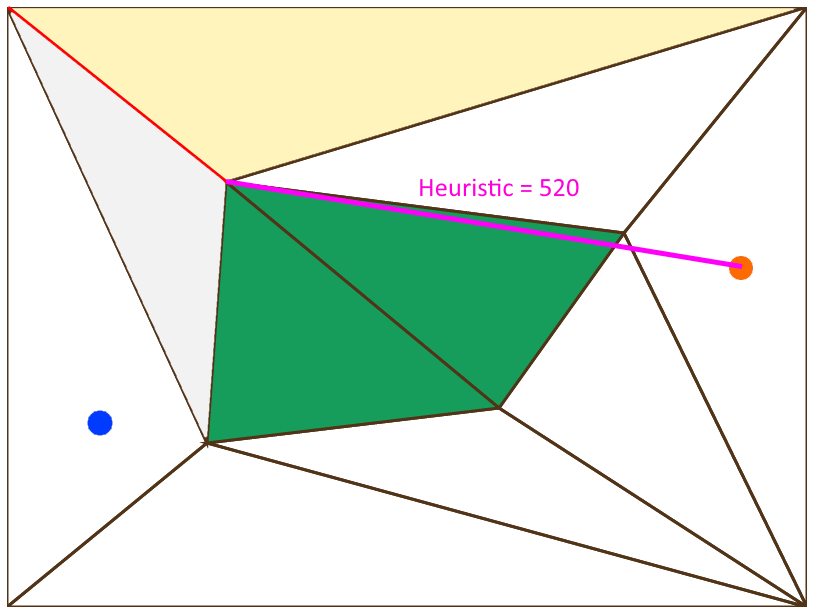The new heuristic calculation is done from the closest point on the entry edge of the triangle to the goal point.

Path length calculation is a bit more difficult, and due to the nature of movement though a triangulated path, has several ways to best estimate the real path length.

A good estimate for the current path length up to a certain triangle is the distance between the starting point of the path and the entry edge (edge between itself and its parent) of the given triangle.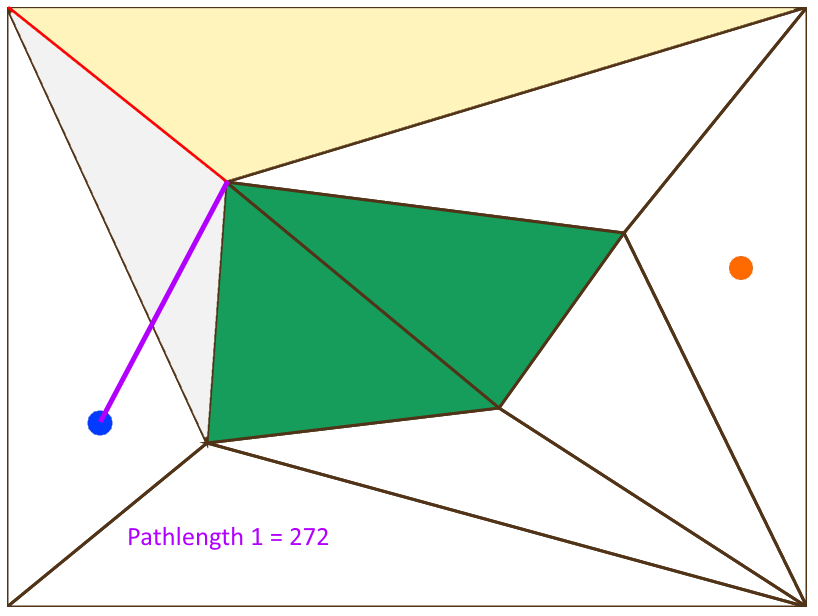A pathlength estimate using the closest point on the entry edge of a triangle and the start point.

Another good estimate for the path length between two triangles is the arc length around their common vertex between the entry edge of each triangle. For a non-zero arc length to be calculated, we need to provide a radius. This should be a value determined by the things we want to follow the path, as their centre-points must remain at least half their width from any vertex when following the path.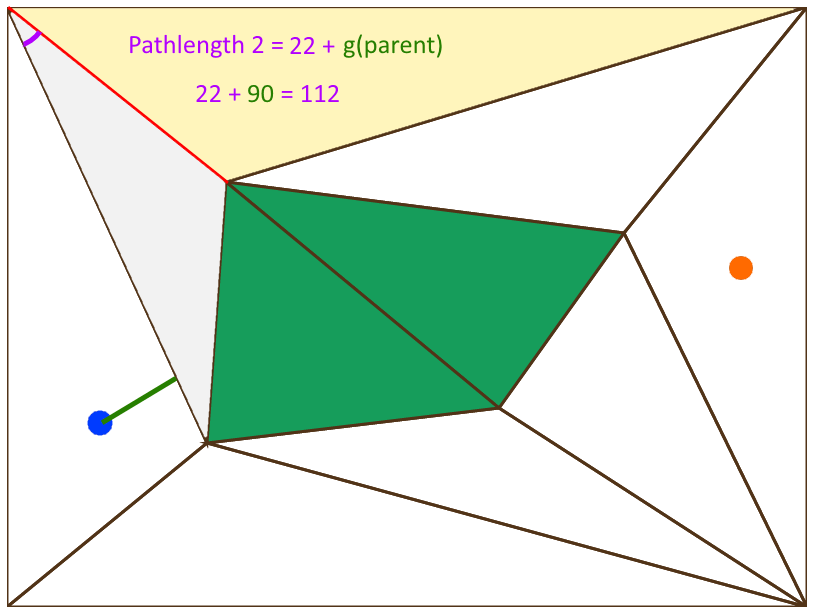A pathlength estimate using the pathlength of the parent plus the arc distance between entry edges with a radius plausible for the moving entities.

Lastly, an estimate path length can be calculated using the heuristic from the child triangle minus the heuristic of the parent triangle.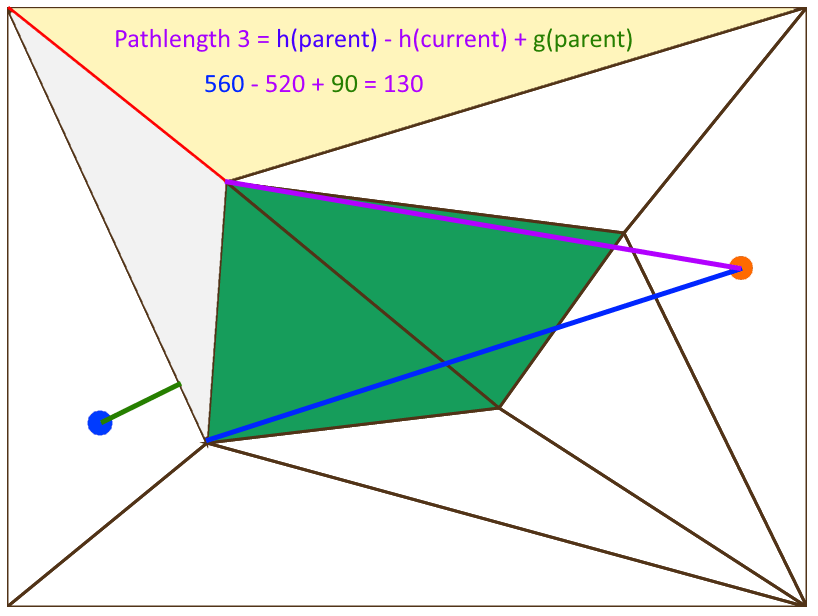A pathlength caculated by subtracting heuristics and adding the current triangle’s parent’s pathlength.

All of these estimates are lower bounds, so using the smallest out of the three would underestimate the true path length. For this reason we use the maximum value of the three estimates and that turns out to provide a reasonably accurate path length.

Given these improvements, more accurate paths are provided over the triangulated area.

In addition to a more accurate path we can further improve how entities follow the path, by reducing the number of things to consider when following the path. A simple way to do this is to think of the path as a series of gateways, theses would be the edges of the triangles the path passes through. From the initial set of gateways we can use some simple math to reduce the number of gateways we will need to consider – to follow the path.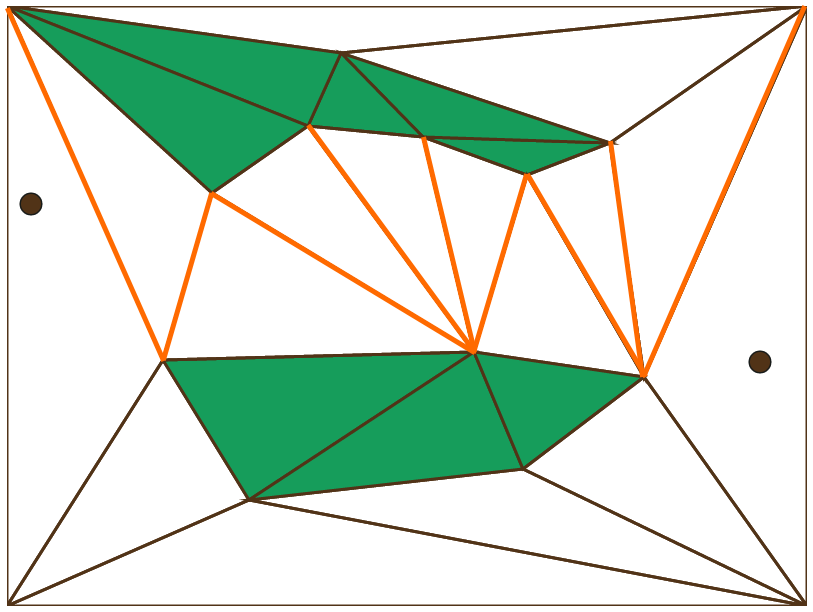The set of gateways the path between the two brown dots would pass through.

This can be done by visualizing a funnel between gateways:

Starting from the first gateway we can define a funnel’s left side between the left edge of the left vertex of the first gateway and the left vertex of the second gateway, we can do the same for the right side. With both sides of the funnel defined, we can then create a secondary funnel between the first gateway and the third.

If this funnel is fully encompassed by the first funnel, we know the first funnel is redundant and can remove the first funnel’s terminal gateway from our path.

If we create a funnel that is not within the previous funnel we made, we can simply restart the process from the last gateway a funnel reached.

This process can be continued until only necessary gateways are leftover. Then when considering where to travel our entities need only consider the next gateway to pass through instead of which triangle to navigate towards.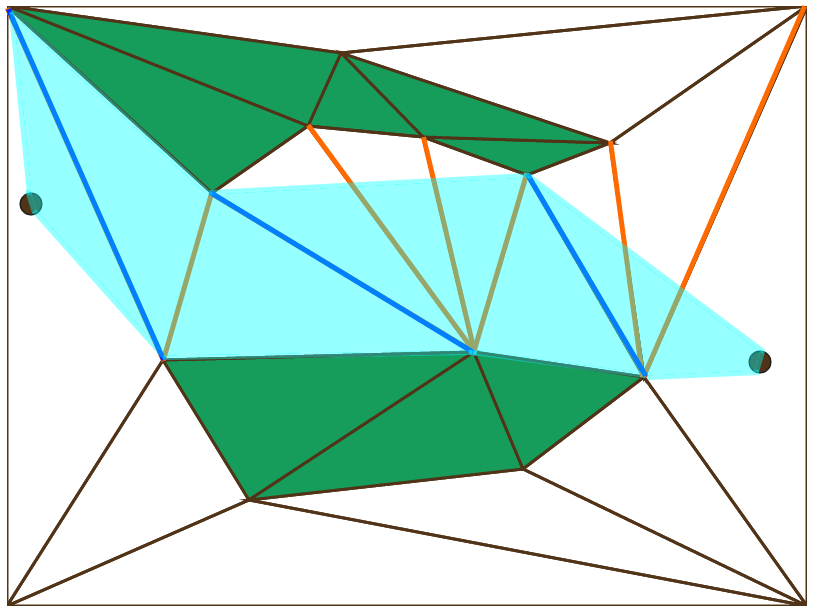The simplified funnel (in light blue) with necessary gateways (in dark blue) for the above set of gateways.

Given this simplified pathway was can now easily navigate the world with a minimal number of re-directions as gateways are passed through. We can also use spline’s or other means to tighten or smooth the path as we now have a simple set of bounds to remain within.

Did you know Raygun supports Unity3D? Enhance your game development and fix bugs faster. Start a free trial of Raygun today.